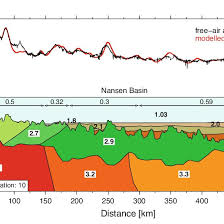## How to Calculate and Solve for Free Air Anomaly | GravityThe image above represents free air anomaly.

To compute for free air anomaly, four essential parameters are needed and these parameters are Observed or Measured Gravity Value (gobs), Reference Value of Gravity (g(λ)), Elevation (h) and Radius of the Earth (R).

The formula for calculating free air anomaly:

δgF = gobs – g(λ) [1 – 2h/R]

Where:
δgF = Free Air Anomaly
gobs = Observed or Measured Gravity Value
g(λ) = Reference Value of Gravity
h = Elevation
R = Radius of the Earth

Let’s solve an example;
Find the free air anomaly when the observed or measured gravity value is 9, reference value of gravity is 10, elevation is 16 and radius of the earth is 4.

This implies that;

gobs = Observed or Measured Gravity Value = 9
g(λ) = Reference Value of Gravity = 10
h = Elevation = 16
R = Radius of the Earth = 4

δgF = gobs – g(λ) [1 – 2h/R]
δgF = 9 – 10 [1 – 2(16)/4]
δgF = 9 – 10 [1 – 32/4]
δgF = 9 – 10 [1 – 8]
δgF = 9 – 10 [-7]
δgF = 9 – -70
δgF = 79

Therefore, the free air anomaly is 79 Gal.